## How Can I Display Confidence Interval like Prediction Limit by PROC SGPLOT?

Hi, All:

I'm making linear regression graph (with xx%CI), and I have trouble changing xx%CI's display.

I want xx%CI graph with dashed line (which expresses confidence limit) like Prediction Limit's display,

but now, I have regression line graph with xx%CI's shaded area by submitting following code.

``````data test;
input x y @@;
datalines;
10 10 4 5 2 5 2 4 8 4 9 6 7 6 5 2
1 1 3 2 4 7 6 7 8 9 11 8 6 4
;
run;
proc sgplot data=test;
reg x=x y=y / cli clm clmattrs=(clmlineattrs=(pattern=dash color=black));
keylegend / noborder location=outside position=top;
run;``````

I tried by using CLMLINEATTRS= option, but I can't understand what changed.

I want xx%CI's display which is expressed by dashed line & no shaded area, and also want to change xx%CI's label 's symbol (from shaded square to dash line).

By using Graphics Designer & following Generated Code, I accomplished my objective.

But I want to accomplish it by PROC SGPLOT, not by PROC TEMPLATE&SGRENDER.

``````ods output outputstatistics=pred(keep=observation predictedvalue lowerclmean upperclmean
rename=(predictedvalue=pred lowerclmean=lower upperclmean=upper));
proc reg data=test;
model y=x / r clm alpha=.1;
run;
quit;
data test_1(drop=observation);
merge test pred;
run;
proc template;
define statgraph sgdesign;
dynamic _HEIGHT _UPPER _LOWER _HEIGHT2 _PREDICT _HEIGHT3 _WEIGHT;
begingraph;
layout lattice / rowdatarange=data columndatarange=data rowgutter=10 columngutter=10;
layout overlay;
bandplot x=_HEIGHT limitupper=_UPPER limitlower=_LOWER / name='band' display=(OUTLINE) connectorder=axis extend=true outlineattrs=(color=CX000000 pattern=MEDIUMDASH );
seriesplot x=_HEIGHT2 y=_PREDICT / name='series' connectorder=xaxis;
scatterplot x=_HEIGHT3 y=_WEIGHT / name='scatter';
discretelegend 'scatter' 'series' 'band' / opaque=false border=false halign=center valign=top displayclipped=true down=1 order=columnmajor location=inside;
endlayout;
endlayout;
endgraph;
end;
run;
proc sgrender data=test_1 template=sgdesign;
dynamic _HEIGHT="x" _UPPER="upper" _LOWER="lower" _HEIGHT2="x" _PREDICT="pred" _HEIGHT3="x" _WEIGHT="y";
run;``````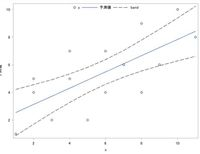Thank you for your kind cooperation.

1 ACCEPTED SOLUTION

Accepted Solutions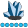SuzanneDorinski
Lapis Lazuli | Level 10

## Re: How Can I Display Confidence Interval like Prediction Limit by PROC SGPLOT?

This code may do what you want.

The documentation for the REG statement includes these two sentences:

CLMLINEATTRS= has no effect unless you change the display options in the style element to display outlines. See the preceding code example.

But I didn't see any preceding code example.  Googling "CLMLINEATTRS=style-element <(options)>" helped me find the code example in the PBSPLINE statement documentation for PROG SGPLOT in the SAS 9.3 ODS Graphics: Procedures Guide, Third Edition.  That's the PROC TEMPLATE below, which is how you turn on the fill outline.

I don't see the code example in the most recent version of the online documentation for the PBSPLINE statement in PROC SGPLOT.

``````proc template;
define style MyStyleDefault;
parent=Styles.htmlblue;
style GraphBand from GraphBand /
DisplayOpts = "Fill outline";
end;
run;

ods html5 (id=web) style= MyStyleDefault;

data test;
input x y @@;
datalines;
10 10 4 5 2 5 2 4 8 4 9 6 7 6 5 2
1 1 3 2 4 7 6 7 8 9 11 8 6 4
;
run;

proc sgplot data=test;
reg x=x y=y / clm clmattrs=(clmfillattrs=(color=white)
clmlineattrs=(pattern=mediumdash color=black thickness=2))
nolegclm;
keylegend "y" "band" "fit" / noborder location=outside position=top;
legenditem type=line name="band" / label="95% Confidence Limits"
lineattrs=(pattern=mediumdash
color=black
thickness=2);
legenditem type=marker name="y" / label="y" markerattrs=(symbol=circle);
legenditem type=line name="fit" / label="Regression" lineattrs=(pattern=solid
thickness=2);
run;``````

The screen shot below is the graphic from the code.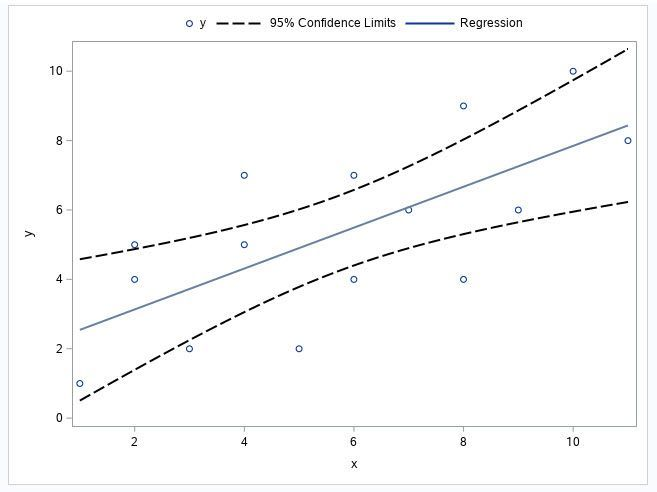Show confidence limit lines in PROC SGPLOT rather than shaded area

4 REPLIES 4SuzanneDorinski
Lapis Lazuli | Level 10

## Re: How Can I Display Confidence Interval like Prediction Limit by PROC SGPLOT?

This code may do what you want.

The documentation for the REG statement includes these two sentences:

CLMLINEATTRS= has no effect unless you change the display options in the style element to display outlines. See the preceding code example.

But I didn't see any preceding code example.  Googling "CLMLINEATTRS=style-element <(options)>" helped me find the code example in the PBSPLINE statement documentation for PROG SGPLOT in the SAS 9.3 ODS Graphics: Procedures Guide, Third Edition.  That's the PROC TEMPLATE below, which is how you turn on the fill outline.

I don't see the code example in the most recent version of the online documentation for the PBSPLINE statement in PROC SGPLOT.

``````proc template;
define style MyStyleDefault;
parent=Styles.htmlblue;
style GraphBand from GraphBand /
DisplayOpts = "Fill outline";
end;
run;

ods html5 (id=web) style= MyStyleDefault;

data test;
input x y @@;
datalines;
10 10 4 5 2 5 2 4 8 4 9 6 7 6 5 2
1 1 3 2 4 7 6 7 8 9 11 8 6 4
;
run;

proc sgplot data=test;
reg x=x y=y / clm clmattrs=(clmfillattrs=(color=white)
clmlineattrs=(pattern=mediumdash color=black thickness=2))
nolegclm;
keylegend "y" "band" "fit" / noborder location=outside position=top;
legenditem type=line name="band" / label="95% Confidence Limits"
lineattrs=(pattern=mediumdash
color=black
thickness=2);
legenditem type=marker name="y" / label="y" markerattrs=(symbol=circle);
legenditem type=line name="fit" / label="Regression" lineattrs=(pattern=solid
thickness=2);
run;``````

The screen shot below is the graphic from the code.Show confidence limit lines in PROC SGPLOT rather than shaded area

## Re: How Can I Display Confidence Interval like Prediction Limit by PROC SGPLOT?

Hi, Suzanne:

But LEGENDITEM statement in PROC SGPLOT applies to SAS9.4M5 or to the latter, and unfortunately, mine is SAS9.4M3.

After I questioned this, I tried another method and got the following "closer" to my objective.

``````proc sgplot data=SASHELP.CLASSFIT;
band x=HEIGHT UPPER=UPPER LOWER=LOWER /  nofill LINEATTRS=(color=CX000000 pattern=SHORTDASH );
series x=HEIGHT y=PREDICT;
scatter x=HEIGHT y=WEIGHT;
run;``````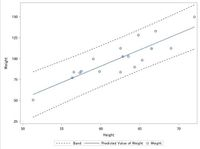It's like my goal and I thought I accomplished, but........

``````data test;
input x y @@;
datalines;
10 10 4 5 2 5 2 4 8 4 9 6 7 6 5 2
1 1 3 2 4 7 6 7 8 9 11 8 6 4
;
run;
ods output outputstatistics=pred(keep=observation predictedvalue lowerclmean upperclmean
rename=(predictedvalue=pred lowerclmean=lower upperclmean=upper));
proc reg data=test;
model y=x / p clm alpha=.1;
run;
quit;
data test_1(drop=observation);
merge test pred;
run;
proc sgplot data=test_1;
reg x=x y=y / clm filledoutlinedmarkers;
run;
proc sgplot data=test_1;
band x=x upper=upper lower=lower / nofill lineattrs=(color=cx000000 pattern=shortdash);
series x=x y=pred;
scatter x=x y=y;
run;``````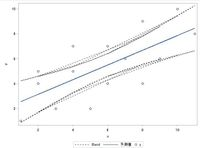I can't understand why multiple CI lines are displayed, and what the difference is between SASHELP.CLASSFIT data & my TEST_1 data.SuzanneDorinski
Lapis Lazuli | Level 10

## Re: How Can I Display Confidence Interval like Prediction Limit by PROC SGPLOT?

The documentation for the BAND statement includes the following note:

 Note: The input data should be sorted by the X or Y variable. If the data is not sorted, the graph might produce unpredictable results.

When I did a sort by X, the graph looked like what you want.  When I did a sort by Y, it didn't look like what you want.

## Re: How Can I Display Confidence Interval like Prediction Limit by PROC SGPLOT?

Hi, Suzanne:

Thank you for your reply & I'm so sorry for my late response.

Like you said, I sorted data by x, and got my wanted.

Thank you so much.

Discussion stats
• 4 replies
• 3871 views
• 2 likes
• 2 in conversation